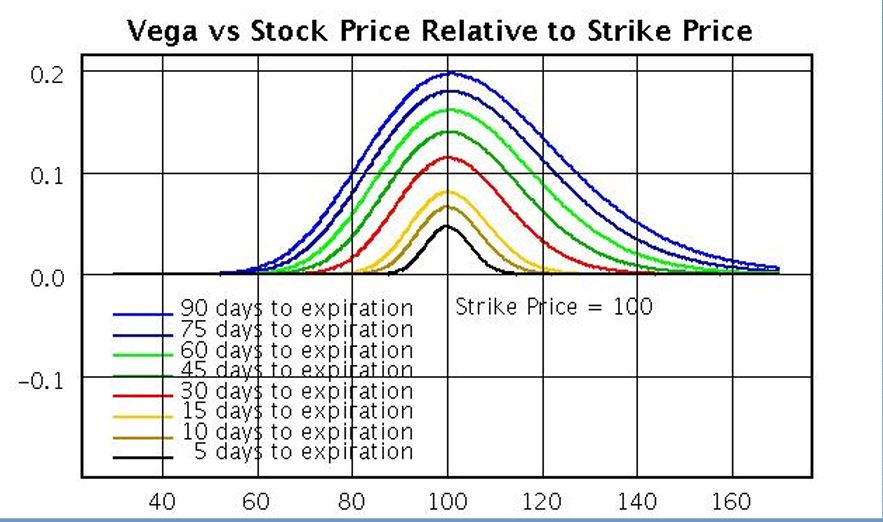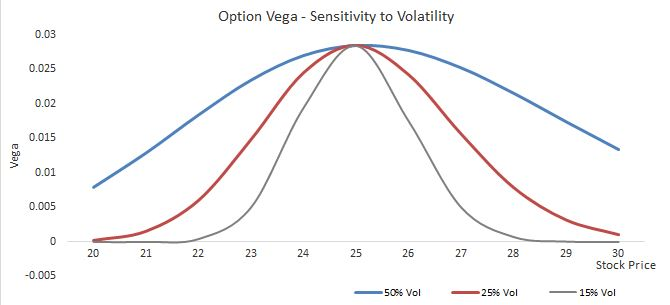Back
BlogsVEGA

RhoW-X

Darren Krett

Monday, 19 December 2022

Share on:Post views: 15404

# VEGA

Categories

The Greeks

Option Vega is a measure of the sensitivity of the price of an option to changes in the implied volatility of the underlying asset. Vega values represent the change in an option’s price given a 1% move in implied volatility, all else equal. This is the option's sensitivity to volatility.Simply put,it describes how the value of an option will change as volatility goes up or down. Option Vega is used by traders to determine potential profits and losses on an option position, especially when volatility changes quickly. Option Vega can also be used to decide when it might be beneficial to adjust a particular position. For example, a trader could increase their exposure with another purchase if implied volatility increases significantly. Conversely, a trader could reduce their exposure by closing out positions if implied volatility falls dramatically.

Vega can also indicate the amount an option's price changes given a 1% change in implied volatility. For example, an option with a Vega of 0.10 indicates the option's value is expected to change by 10 cents if the implied volatility changes by 1%.

Calculating Option Vega for a given position requires a few steps. First, you need to determine the price of the option in question as well as its current implied volatility. Next, you need to calculate the “vega per one-point increase (or decrease) in implied volatility”, by subtracting the original option price from the new one and dividing it by the amount of change in implied volatility. Finally, multiply this result by 100 (since vega is measured in terms of 0.01 of volatility). This will give you the option vega for that particular option position.No attachments

RhoW-X

The GreeksDarren Krett

Monday, 19 December 2022

## Beta

Beta is the greek that allows us to weight our current positions with a designated benchmark.
Beta

0